# 13. Statistical Analysis in SAS¶

Now we are going to cover how to perform a variety of basic statistical tests in SAS.

• Proportion tests

• Chi-squared

• Fisher’s Exact Test

• Correlation

• T-tests/Rank-sum tests

• One-way ANOVA/Kruskal-Wallis

• Linear Regression

• Logistic Regression

• Poisson Regression

Note: We will be glossing over the statistical theory and “formulas” for these tests. There are plenty of resources online for learning more about these tests if you have not had a course covering this material. You will only be required to write code to fit or perform these test but will not be expected to interpret the results for this course.

## 13.1. Proportion Tests¶

To conduct a test for one proportion, we can use PROC FREQ. To get this test, we use the BINOMIAL option in the TABLES statement. As options to BINOMIAL, we can specify

• p= - the null value for the hypothesis test

• level= - which group to use as a “success”

• CORRECT - uses a continuity correction for calculating the p-value (can be useful for small sample sizes)

• CL= - can select different types of CI such as WALD, EXACT, and LOGIT.

### Example

In the following example, we use a summarized dataset, where we have the counts of the "successes" and "failures". In this case, we are interested in the proportion of smokers, so we have a count of smokers and a count of non-smokers.

DATA smoke;
INPUT smkstatus $count; DATALINES; Y 15 N 17 ; RUN; PROC FREQ data = smoke; TABLES smkstatus / binomial(p = 0.5 level = "Y" CORRECT) alpha = 0.05; WEIGHT count; RUN;  SAS Output The SAS System The FREQ Procedure smkstatus Frequency Percent Cumulative Frequency Cumulative Percent N 17 53.13 17 53.13 Y 15 46.88 32 100.00 Binomial Proportion smkstatus = Y Proportion 0.4688 ASE 0.0882 95% Lower Conf Limit 0.2802 95% Upper Conf Limit 0.6573 Exact Conf Limits 95% Lower Conf Limit 0.2909 95% Upper Conf Limit 0.6526 Test of H0: Proportion = 0.5 ASE under H0 0.0884 Z -0.1768 One-sided Pr < Z 0.4298 Two-sided Pr > |Z| 0.8597 Sample Size = 32 Note the use of the WEIGHT statement to specify the counts for Y and N. Without this statement SAS would read our data as having 1 Y and 1 N. The estimated proportion is 0.4688. The (asymptotic) 95% CI is (0.2802, 0.6573) and the two sided (continuity corrected) p-value for testing$H_0: p=0.5$vs$H_a: p\neq 0.5\$ is 0.8597.

Alternatively, we could have had the data listed out for each individual as follows.

DATA smoke2;
DO i = 1 to 15;
smkstatus = "Y";
OUTPUT;
END;
DO i = 1 to 17;
smkstatus = "N";
OUTPUT;
END;
DROP i;
RUN;

PROC FREQ data = smoke2;
TABLES smkstatus / binomial(p = 0.5 level = "Y" CORRECT) alpha = 0.05;
RUN;

SAS Output

The SAS System

The FREQ Procedure

smkstatus Frequency Percent Cumulative
Frequency
Cumulative
Percent
N 17 53.13 17 53.13
Y 15 46.88 32 100.00
Binomial Proportion
smkstatus = Y
Proportion 0.4688
ASE 0.0882
95% Lower Conf Limit 0.2802
95% Upper Conf Limit 0.6573

Exact Conf Limits
95% Lower Conf Limit 0.2909
95% Upper Conf Limit 0.6526
Test of H0: Proportion = 0.5
ASE under H0 0.0884
Z -0.1768
One-sided Pr < Z 0.4298
Two-sided Pr > |Z| 0.8597

Sample Size = 32

## 13.2. Chi-squared Test¶

To test for an association between two categorical variables, we could perform a chi-square test of independence. Again, we will use PROC FREQ with a tables statement. For 2x2 tables, a chi-square test is automatically performed, but for larger tables, we can request is by providing the CHISQ option to the tables statement. Another useful option to also specify is the EXPECTED option which provided the expected cell counts under the null hypothesis of independence. These expected cell counts are needed to assess whether or not the chi-square test is appropriate.

### Example

The following example uses the Kaggle car auction dataset to test for an association between online sales and a car being a bad buy.

FILENAME cardata '/folders/myfolders/SAS_Notes/data/kaggleCarAuction.csv';

PROC IMPORT datafile = cardata out = cars dbms = CSV replace;
getnames = yes;
guessingrows = 1000;
RUN;

PROC FREQ data = cars;
RUN;

SAS Output

The SAS System

The FREQ Procedure

Frequency
Expected
Percent
Row Pct
Col Pct
0 1 Total
0
62375
62389
85.47
97.45
87.68
1632
1618.1
2.24
2.55
88.46
64007

87.70

1
8763
8749.1
12.01
97.63
12.32
213
226.91
0.29
2.37
11.54
8976

12.30

Total
71138
97.47
1845
2.53
72983
100.00

Statistic DF Value Prob
Chi-Square 1 0.9978 0.3178
Likelihood Ratio Chi-Square 1 1.0154 0.3136
Continuity Adj. Chi-Square 1 0.9274 0.3356
Mantel-Haenszel Chi-Square 1 0.9978 0.3179
Phi Coefficient   -0.0037
Contingency Coefficient   0.0037
Cramer's V   -0.0037
Fisher's Exact Test
Cell (1,1) Frequency (F) 62375
Left-sided Pr <= F 0.1679
Right-sided Pr >= F 0.8498

Table Probability (P) 0.0177
Two-sided Pr <= P 0.3324

Sample Size = 72983

The chi-square test results in a p-value of 0.3178, or if we use the chi-square test with continuity correction, then we get a p-value of 0.3356.

In the 2x2 case, as in this example, we may also want measures of effert such as the risk difference, relative risk and odds ratio. We can obtain these using the RISKDIFF, RELRISK, and OR options which will request all three measures with confidence intervals.

PROC FREQ data = cars;
RUN;

SAS Output

The SAS System

The FREQ Procedure

Frequency
Percent
Row Pct
Col Pct
0 1 Total
0
62375
85.47
97.45
87.68
1632
2.24
2.55
88.46
64007
87.70

1
8763
12.01
97.63
12.32
213
0.29
2.37
11.54
8976
12.30

Total
71138
97.47
1845
2.53
72983
100.00

Column 1 Risk Estimates
Risk ASE 95%
Confidence Limits
Exact 95%
Confidence Limits
Row 1 0.9745 0.0006 0.9733 0.9757 0.9733 0.9757
Row 2 0.9763 0.0016 0.9731 0.9794 0.9729 0.9793
Total 0.9747 0.0006 0.9736 0.9759 0.9736 0.9758
Difference -0.0018 0.0017 -0.0051 0.0016
Column 2 Risk Estimates
Risk ASE 95%
Confidence Limits
Exact 95%
Confidence Limits
Row 1 0.0255 0.0006 0.0243 0.0267 0.0243 0.0267
Row 2 0.0237 0.0016 0.0206 0.0269 0.0207 0.0271
Total 0.0253 0.0006 0.0241 0.0264 0.0242 0.0264
Difference 0.0018 0.0017 -0.0016 0.0051
Odds Ratio and Relative Risks
Statistic Value 95% Confidence Limits
Odds Ratio 0.9290 0.8040 1.0735
Relative Risk (Column 1) 0.9982 0.9947 1.0016
Relative Risk (Column 2) 1.0745 0.9331 1.2373

Sample Size = 72983

For the risk difference, SAS provides two tables that compare the conditional row proportions in the first column and the conditional row proportions in the second column. Similarly, for the relative risk, we get a relative risk for the first and the second column. This allows us to pick the one that matters to us depending on which column corresponds to the outcome of interest.

## 13.3. Fisher’s Exact Test¶

An alternative way to test for an association between two categorical variables is Fisher’s exact test. This test is a nonparametric test that makes no assumption other than that we have a random sample. Note, however, that this comes with a price. The more levels our variables have and the more observations we have will increase the computing time needed to perform this test. For 2x2 tables, this test is usally very quick, but for 5x5 tables, depending on how much data and what computer you are using, this test may take hours to complete.

For 2x2 tables, this test is automatically output. For larger tables, if you want this test, then you will need to specify the FISHER option in the TABLES statement.

### Example

The following SAS program uses Fisher's exact test to test for an association between a car being a bad buy and buying the car online.

PROC FREQ data = cars;
RUN;

SAS Output

The SAS System

The FREQ Procedure

Frequency
Percent
Row Pct
Col Pct
0 1 Total
0
62375
85.47
97.45
87.68
1632
2.24
2.55
88.46
64007
87.70

1
8763
12.01
97.63
12.32
213
0.29
2.37
11.54
8976
12.30

Total
71138
97.47
1845
2.53
72983
100.00

Statistic DF Value Prob
Chi-Square 1 0.9978 0.3178
Likelihood Ratio Chi-Square 1 1.0154 0.3136
Continuity Adj. Chi-Square 1 0.9274 0.3356
Mantel-Haenszel Chi-Square 1 0.9978 0.3179
Phi Coefficient   -0.0037
Contingency Coefficient   0.0037
Cramer's V   -0.0037
Fisher's Exact Test
Cell (1,1) Frequency (F) 62375
Left-sided Pr <= F 0.1679
Right-sided Pr >= F 0.8498

Table Probability (P) 0.0177
Two-sided Pr <= P 0.3324

Sample Size = 72983

The p-value for Fisher's exact test is 0.3324.

## 13.4. Correlation¶

SAS’s CORR procedure can perform correlation analysis by providing both the parametric Pearson’s correlation and the nonparametric Spearman’s rank correlation coefficients and hypothesis tests. The default correlation output is Pearson’s. To request the Spearman’s rank correlation, add the SPREAMAN option to the PROC CORR statement.

### Example

Let's look at some examples using PROC CORR using the Charm City Circulator bus ridership dataset. The following SAS program will find the Pearson correlation and hypothesis test results for the correlation between the average daily ridership between the orange and purple bus lines.

FILENAME busdata '/folders/myfolders/SAS_Notes/data/Charm_City_Circulator_Ridership.csv';

PROC IMPORT datafile = busdata out = circ dbms = CSV replace;
getnames = yes;
guessingrows = 1000;
RUN;

PROC CORR data = circ;
VAR orangeAverage purpleAverage;
RUN;

SAS Output

The SAS System

The CORR Procedure

2 Variables: orangeAverage purpleAverage
Simple Statistics
Variable N Mean Std Dev Sum Minimum Maximum
orangeAverage 1136 3033 1228 3445671 0 6927
purpleAverage 993 4017 1407 3988816 0 8090
Pearson Correlation Coefficients
Prob > |r| under H0: Rho=0
Number of Observations
orangeAverage purpleAverage
orangeAverage
1.00000

1136
0.91954
<.0001
993
purpleAverage
0.91954
<.0001
993
1.00000

993

### Example

We can also get a correlation matrix for multiple variables at the same time. The following example also uses the NOMISS option to only use complete observations instead of pairwise complete observations when calculating the correlations. Here we get the correlation matrix between average ridership counts between all four of the orange, purple, banner, and green bus lines.

PROC CORR data = circ NOMISS;
VAR orangeAverage purpleAverage greenAverage bannerAverage;
RUN;

SAS Output

The SAS System

The CORR Procedure

4 Variables: orangeAverage purpleAverage greenAverage bannerAverage
Simple Statistics
Variable N Mean Std Dev Sum Minimum Maximum
orangeAverage 270 3859 1095 1041890 0 6927
purpleAverage 270 4552 1297 1228935 0 8090
greenAverage 270 2090 556.00353 564213 0 3879
bannerAverage 270 827.26852 436.04872 223363 0 4617
Pearson Correlation Coefficients, N = 270
Prob > |r| under H0: Rho=0
orangeAverage purpleAverage greenAverage bannerAverage
orangeAverage
1.00000

0.90788
<.0001
0.83958
<.0001
0.54470
<.0001
purpleAverage
0.90788
<.0001
1.00000

0.86656
<.0001
0.52135
<.0001
greenAverage
0.83958
<.0001
0.86656
<.0001
1.00000

0.45334
<.0001
bannerAverage
0.54470
<.0001
0.52135
<.0001
0.45334
<.0001
1.00000

If we don't want all pairwise correlations, but instead only specific pairs, then we can use the WITH statement as in the following example.

PROC CORR data = circ NOMISS;
VAR orangeAverage purpleAverage;
WITH greenAverage bannerAverage;
RUN;

SAS Output

The SAS System

The CORR Procedure

2 With Variables: greenAverage bannerAverage orangeAverage purpleAverage
Simple Statistics
Variable N Mean Std Dev Sum Minimum Maximum
greenAverage 270 2090 556.00353 564213 0 3879
bannerAverage 270 827.26852 436.04872 223363 0 4617
orangeAverage 270 3859 1095 1041890 0 6927
purpleAverage 270 4552 1297 1228935 0 8090
Pearson Correlation Coefficients, N = 270
Prob > |r| under H0: Rho=0
orangeAverage purpleAverage
greenAverage
0.83958
<.0001
0.86656
<.0001
bannerAverage
0.54470
<.0001
0.52135
<.0001

To get Spearman’s rank correlation instead of Pearson’s correlation, add the SPEARMAN option to the PROC CORR statement.

### Example

The following SAS program produces Spearman's rank correlation coefficient and associated p-value for the hypothesis test of the correlation is 0 between the average daily ridership counts betwen the orange and purple bus lines.

PROC CORR data = circ SPEARMAN;
VAR orangeAverage purpleAverage;
RUN;

SAS Output

The SAS System

The CORR Procedure

2 Variables: orangeAverage purpleAverage
Simple Statistics
Variable N Mean Std Dev Median Minimum Maximum
orangeAverage 1136 3033 1228 2968 0 6927
purpleAverage 993 4017 1407 4223 0 8090
Spearman Correlation Coefficients
Prob > |r| under H0: Rho=0
Number of Observations
orangeAverage purpleAverage
orangeAverage
1.00000

1136
0.91455
<.0001
993
purpleAverage
0.91455
<.0001
993
1.00000

993

## 13.5. T-Tests¶

T-tests can be performed in SAS with the TTEST procedure including

• one sample t-test

• paired t-test

• Two sample t-test

### Example

In this example, we will test if the average daily ridership on the orange bus line is greater than 3000 using a one sample t-test.

PROC TTEST data = circ H0 = 3000 SIDE = U;
VAR orangeAverage;
RUN;

SAS Output

The SAS System

The TTEST Procedure

Variable: orangeAverage

N Mean Std Dev Std Err Minimum Maximum
1136 3033.2 1227.6 36.4217 0 6926.5
Mean 95% CL Mean Std Dev 95% CL Std Dev
3033.2 2973.2 Infty 1227.6 1179.1 1280.3
DF t Value Pr > t
1135 0.91 0.1814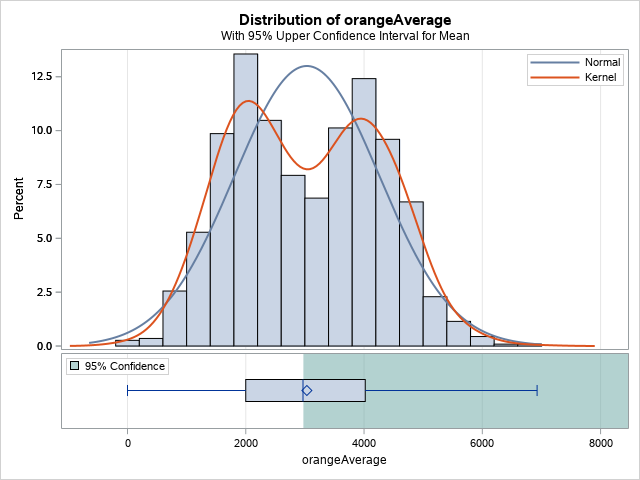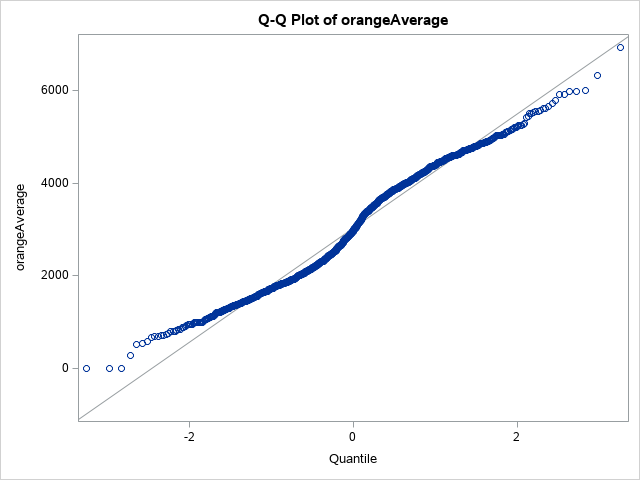The H0= option specifies the null value in the t-test and the SIDE= option specifies whether you want a less than (L), greater than (U), or not equal to (2) test. The default values are 0 for the null hypothesis value and two sided (2) for the alternative hypothesis. The output provides some summary statistics, the p-value for the test, confidence interval and a histogram and QQ plot to assess the normality assumption.

From the output, we find the p-value to be 0.1814. Since we requested a one-side test, we get a one-sided confidence interval. To get our usual (two-sided) confidence interval, we need to request a two-sided test.

For a two sample t-test, we need to have the data formatted in two columns:

• A data column that contains the quantitative data for both groups

• A grouping variable column that indicates the group for the data value in that row.

In PROC TTEST, we put the data variable in the VAR statement and the grouping variable in the CLASS statement to get a two sample t-test.

### Example

In the following SAS program, we perform a two-sample t-test between the orange and purple bus lines' average ridership counts. We will first have to transform the data to meet the required data format for PROC TTEST.

DATA circ_sub;
SET circ;
count = orangeAverage;
group = "orange";
OUTPUT;
count = purpleAverage;
group = "purple";
OUTPUT;
KEEP count group;
RUN;

PROC TTEST data = circ_sub;
VAR count;
CLASS group;
RUN;

SAS Output

The SAS System

The TTEST Procedure

Variable: count

group Method N Mean Std Dev Std Err Minimum Maximum
orange   1136 3033.2 1227.6 36.4217 0 6926.5
purple   993 4016.9 1406.7 44.6388 0 8089.5
Diff (1-2) Pooled   -983.8 1314.1 57.0906
Diff (1-2) Satterthwaite   -983.8   57.6122
group Method Mean 95% CL Mean Std Dev 95% CL Std Dev
orange   3033.2 2961.7 3104.6 1227.6 1179.1 1280.3
purple   4016.9 3929.3 4104.5 1406.7 1347.4 1471.4
Diff (1-2) Pooled -983.8 -1095.7 -871.8 1314.1 1275.8 1354.9
Diff (1-2) Satterthwaite -983.8 -1096.8 -870.8
Method Variances DF t Value Pr > |t|
Pooled Equal 2127 -17.23 <.0001
Satterthwaite Unequal 1984 -17.08 <.0001
Equality of Variances
Method Num DF Den DF F Value Pr > F
Folded F 992 1135 1.31 <.0001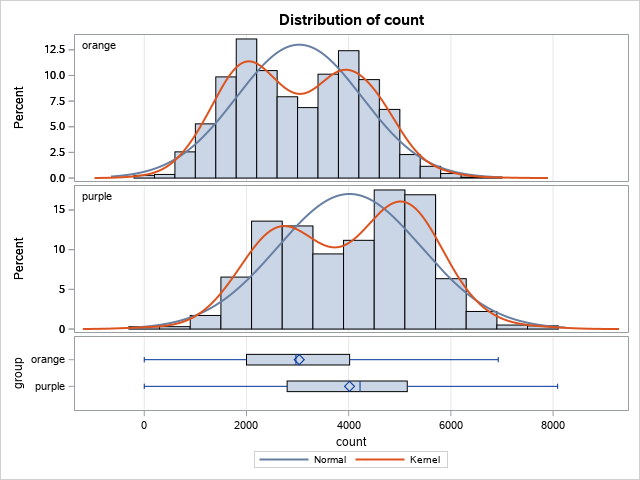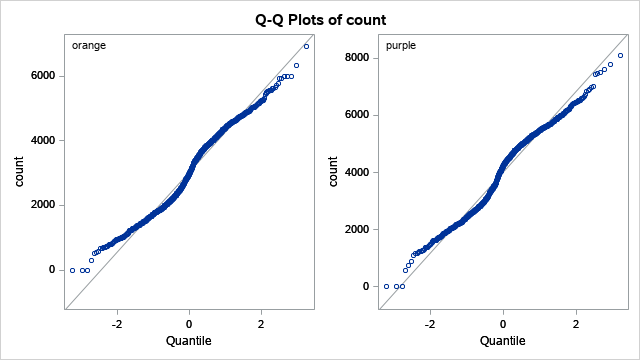The SAS output contains summary statistics for each group, confidence intervals for each group mean, confidence intervals for the difference of the two means, hypothesis tests for the difference of the two means, and the F test for equality of variances. The Pooled row corresponds to the two sample t-test which assumes the population variances are equal between the two groups while the Satterthwaite assumes that the population variances are unequal.

Note that the data here are really matched pairs data, since we have average ridership counts matched by date between the two bus lines. We will explore the paired t-test next.

To perform a paired t-test, we need to use the PAIRED statement. In this case, SAS assumes the data from each group are in two separate columns where observations in the same row correspond to the matched pairs.

### Example

The following SAS program performs a paired t-test betwen the average ridership counts between the orange and purple bus lines.

PROC TTEST data = circ;
PAIRED orangeAverage*purpleAverage;
RUN;

SAS Output

The SAS System

The TTEST Procedure

Difference: orangeAverage - purpleAverage

N Mean Std Dev Std Err Minimum Maximum
993 -764.1 572.3 18.1613 -2998.0 2504.5
Mean 95% CL Mean Std Dev 95% CL Std Dev
-764.1 -799.8 -728.5 572.3 548.2 598.6
DF t Value Pr > |t|
992 -42.08 <.0001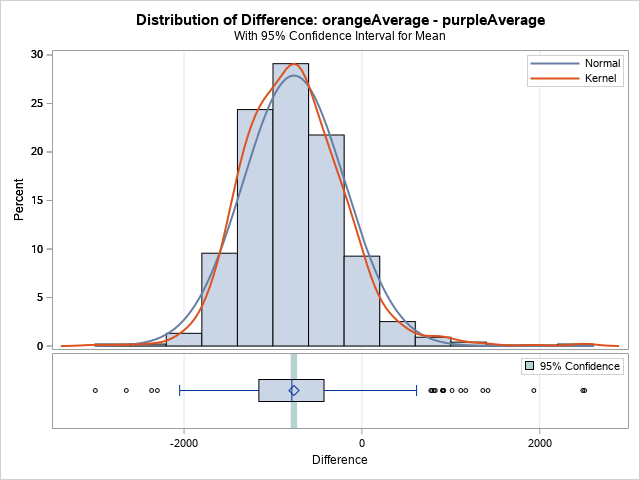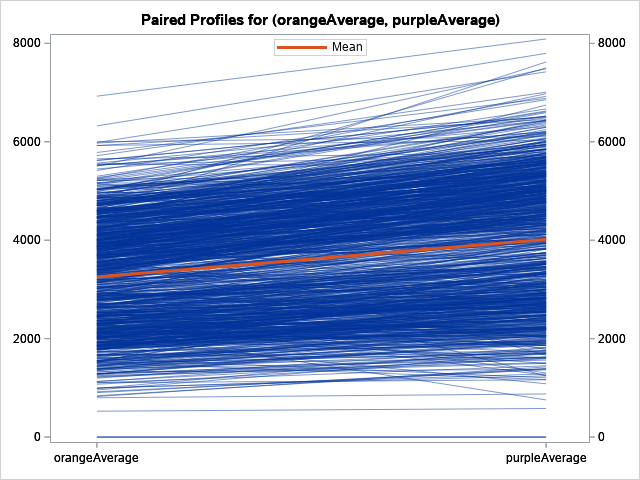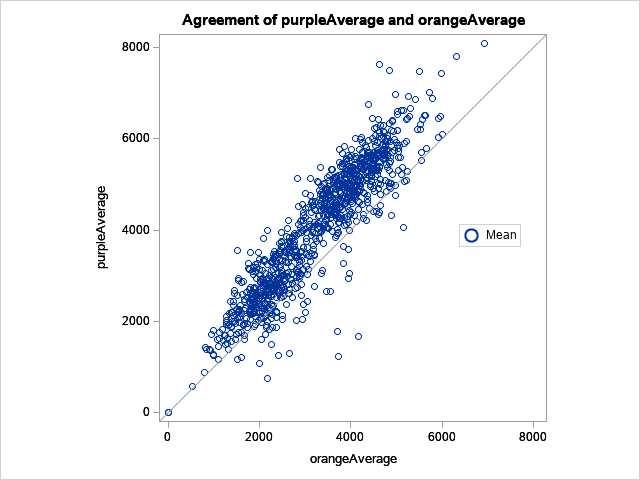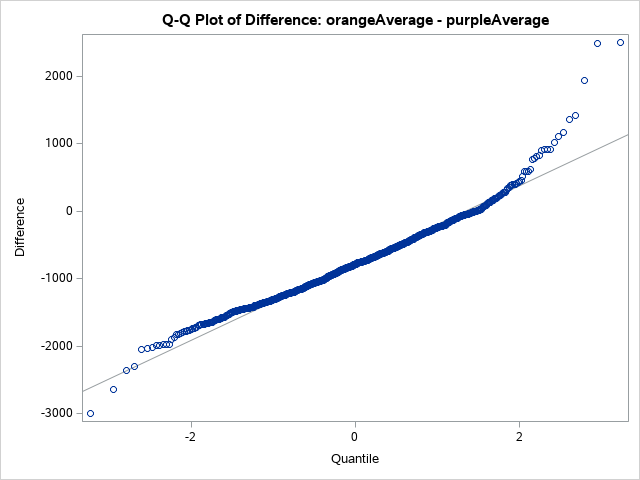## 13.6. Nonparametric Alternatives to the T-Tests¶

In the case that we have a small sample size and the data cannot be assumed to be from populations that are Normally distributed, we need to use a nonparametric test. For the t-tests we have the following possible alternative tests:

• The sign test or the Wilcoxon signed rank test as alternative to the one sample t-test or the paired t-test.

• The Wilcoxon rank sum test as an alternative to the two sample t-test.

To perform a Wilcoxon rank sum test, we use PROC NPAR1WAY.

### Example

In the following example, we use PROC NPAR1WAY to perform Wilcoxon rank sum test to compare median daily ridership counts between the orange and purple bus lines.

PROC NPAR1WAY data = circ_sub WILCOXON;
VAR count;
CLASS group;
RUN;

SAS Output

The SAS System

The NPAR1WAY Procedure

Wilcoxon Scores (Rank Sums) for Variable count
Classified by Variable group
group N Sum of
Scores
Expected
Under H0
Std Dev
Under H0
Mean
Score
orange 1136 982529.50 1209840.0 14150.2115 864.90273
purple 993 1284855.50 1057545.0 14150.2115 1293.91289
Wilcoxon Two-Sample Test
Statistic Z Pr > Z Pr > |Z| t Approximation
Pr > Z Pr > |Z|
1284856 16.0641 <.0001 <.0001 <.0001 <.0001
Kruskal-Wallis Test
Chi-Square DF Pr > ChiSq
258.0555 1 <.0001